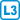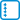## Game with dice

A strange character on the street asks you to play a gambling game with dice according to the following rules. The game is played with three dice, each of which has five empty faces and on the remaining face is a number: the first die has 7, the second has 11 and the third has 13. If you bet 20 crowns, you will win a number of crowns determined by the product of the numbers that you see after throwing the dice – in the best case over a thousand crowns. More often, however, you will win nothing, which happens when all three dice fall with empty faces upward.

The required bet of 20 crowns seemed too large to you, so you quickly talked him down to 16 crowns, and after a long negotation down to 15.5 crowns.

Who would earn money if you played this game for a long time – you or the gambler? (Assume that the dice are fair, and so on.)

• #### Solution

We will first change the game slightly and imagine that there are ones on the empty faces.

We introduce three random variables corresponding to the average values on each of the three dice. We can easily compute that the first variable has expected value 2, the second $$\frac83$$ and the third 3. These random variables are independent, so the expected payout in the modified game is $$2 \cdot \frac83 {\cdot} 3=16$$. It might appear that if we can negotiate the required bet to be less than 16 crowns, we can win in the long term.

However in the original game the other sides of the dice were empty and did not have ones. So we must subtract the payouts when only ones are thrown. This event has probability $$\left(\frac{5}{6}\right)^3=\frac{125}{216}$$ and we must reduce the expected value of the payout by this amount (think about why).

The expected payout in the original game is $$16-\frac{125}{216}\doteq 15{,}42<15{,}5$$.